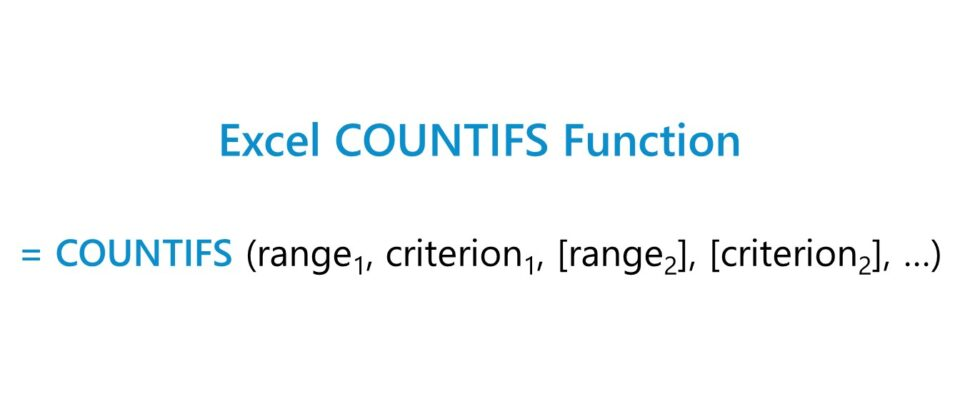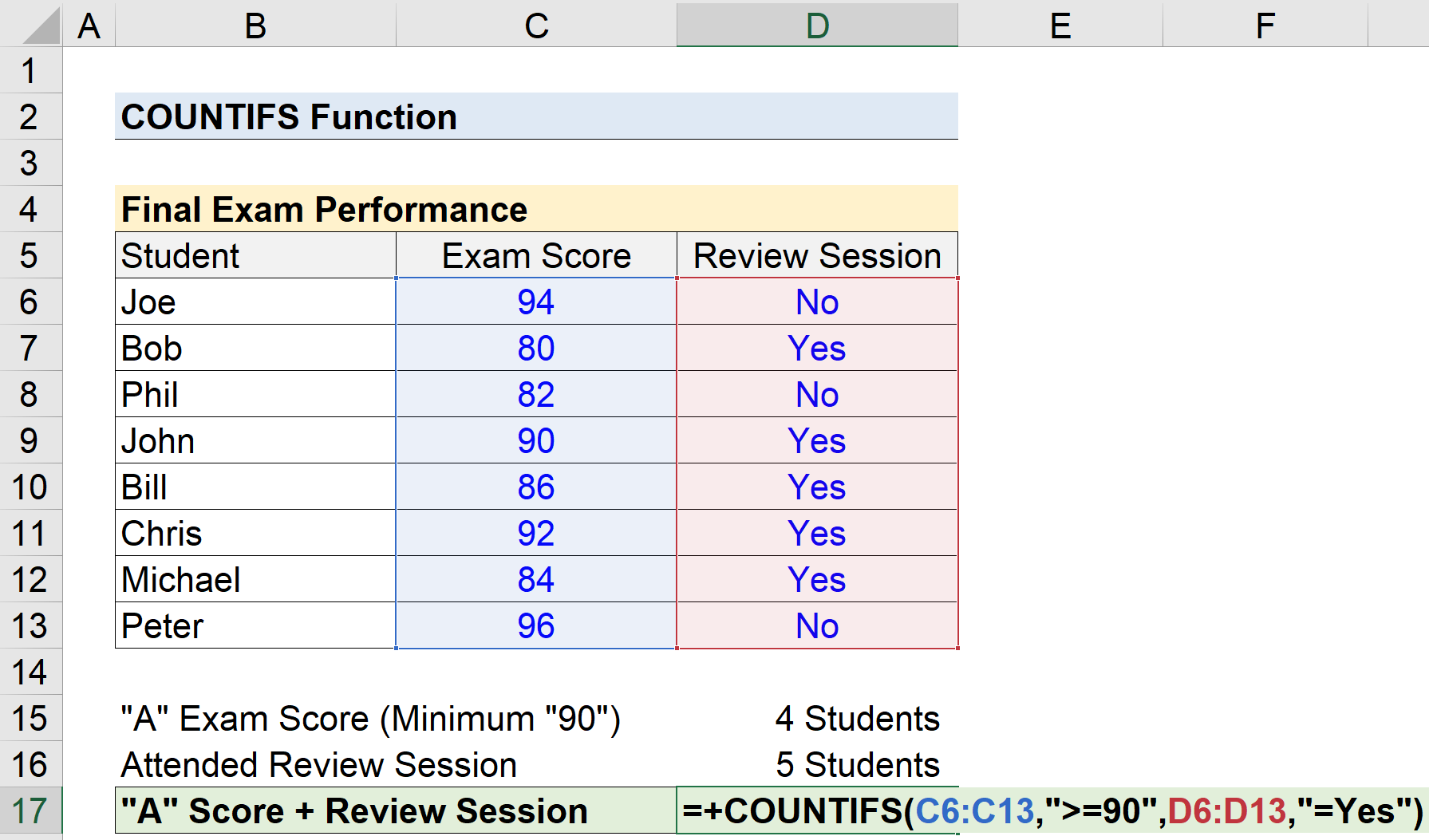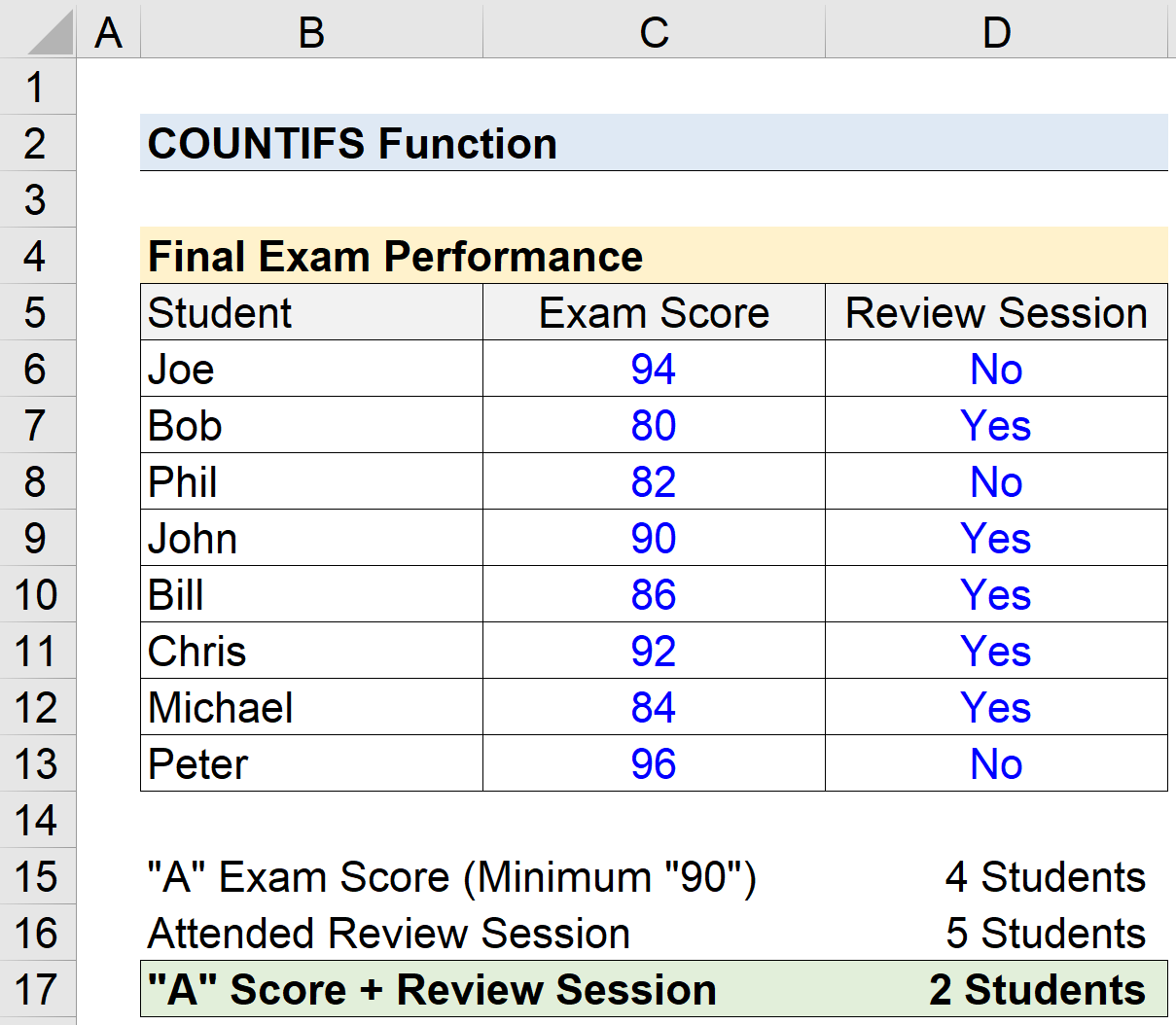Welcome to Wall Street Prep! Use code at checkout for 15% off.# COUNTIFS Function

Guide to Understanding the COUNTIFS Function in Excel## How to Use COUNTIFS Function in Excel (Step-by-Step)

The Excel “COUNTIFS” function is used to count the number of cells in a selected range that meets multiple conditions as specified by the user.

Given set criteria, i.e. the set conditions that must be met, the COUNTIFS function in Excel counts the cells that fulfill those conditions.

For example, the user could be a professor looking to count the number of students that received an “A” score on a final exam, of the students that attended a review session held before the exam.

## Excel COUNTIFS vs. COUNTIF: What is the Difference?

In Excel, the COUNTIFS function is an extension of the “COUNTIF” function.

• COUNTIF Function → While the COUNTIF function is useful for counting the number of cells that meet certain criteria, the user is constrained to only one condition.
• COUNTIFS Function → In contrast, the COUNTIFS function supports multiple conditions, thereby making it more practical due to its broadened scope.

## Excel COUNTIFS Function Formula Syntax

The formula for using the COUNTIFS function in Excel is as follows.

=COUNTIFS(range1, criterion1, [range2], [criterion2], …)
• “range” → The selected range of data that the function will count the cells within that match the stated criterion.
• “criterion” → The specific condition that must be met to be counted by the function.

After the initial two range and criterion inputs, the rest have brackets surrounding them, which are meant to denote that those are optional inputs and can be left blank, i.e. “omitted”.

Unique to the COUNTIFS function, the underlying logic is based on an “AND” criteria, meaning that all the conditions listed must be met for a cell to be “counted”.

Said differently, if a cell meets one condition, yet fails to meet the second condition, the cell will NOT be counted.

For those wanting to use the “OR” logic instead, multiple separate COUNTIFS functions can be used and then added together.

## Text Strings and Numeric Criterion

The selected range can consist of text strings such as the name of a city (e.g. Dallas), as well as a number like the population of the city (e.g. 1,325,691).

The most commonly used examples of logical operators are the following:

Logical Operator Description
=
• “Equal to”
>
• “Greater than”
<
• “Less than”
>=
• “Greater than or Equal to”
<=
• “Less than or Equal to”
<>
• “Not Equal To”

## Date, Text and Blank and Non-Blank Conditions

In order for a logical operator to function properly, it is necessary to enclose the operator and criterion in double quotes, otherwise the formula will not work.

There are exceptions, however, such as a numeric criterion where the user is looking for a specific number (e.g. =20).

In addition, text strings containing binary conditions such as “True” or “False” are not required to be enclosed in parentheses.

Criterion Type Description
Text
• The criterion type can be related to containing certain text, such as the name of a person, city, country, etc.
Date
• The criterion type could be related to specific dates, where the function count the entries based on the logical operator.
Blank Cells
• The double quote (””) counts the number of blank cells in the selected range.
Non-Blank Cells
• The ”<>” operator counts the number of non-blank cells, i.e. any cell containing a number, text, date, or cell reference is counted.
Cell References
• The criterion can contain a cell references as well (e.g. A1). However, the cell reference itself should not be enclosed in quotes. For instance, the proper format if counting cells equal to cell A1 would be “=”&A1.

## Wildcards in COUNTIFS

Wildcards are a term that refers to special characters such as a question mark (?), asterisk (*), and tilde (~) in the criterion.

Wildcard Description
(?)
• The question mark in the criteria matches any single character.
(*)
• The asterisk in the criteria matches zero (or more) characters of any sort, in order to count cells that contain a specific word. For example, “*TXwill count any cell that ends in “TX”.
(~)
• The tilde matches a wildcard, e.g. “~?” counts any cells that end with a question mark.

## COUNTIFS Function Calculator – Excel Model Template

We’ll now move on to a modeling exercise, which you can access by filling out the form below.Submitting...

## Excel COUNTIFS Function Calculation Example

Suppose we’re given the following data on a classroom’s final exam performance.

Our task is to count the number of students that received a score of an “A” on a final exam, i.e. greater than or equal to a score of 90%, that also attended the review session prior to the exam date.

The left column contains the names of the students in the class, while the two columns to the right state the grade received by the student and the status of review session attendance (i.e. either “Yes” or “No”).

Student Final Exam Grade Review Session Attendance
Joe 94 Yes
Bob 80 No
Phil 82 No
John 90 Yes
Bill 86 Yes
Chris 92 Yes
Michael 84 No
Peter 96 Yes

Our goal here is to evaluate the effectiveness of the review session to see if there is a notable correlation between two factors:

1. Review Session Attendance
2. Earning a Minimum Grade of 90% (“A”)

With that said, we’ll begin by counting the number of students that earned an “A”, followed by the number of students that attended the review session.

The COUNTIF function can be used to calculate each figure, since there is only one condition each.

=COUNTIF(C6:C13,”>=90″)
=COUNTIF(D6:D13,”=Yes”)

Of the ten students in the class, we’ve determined that 4 students earned a final exam grade either greater than or equal to 90, while five students attended the final exam review session.

In the final part, we’ll use the COUNTIFS function to determine the number of students that received an “A” exam grade and attended the review session.

=COUNTIFS(C6:C13,”>=90″,D6:D13,”=Yes”)Using the COUNTIFS function, we’ve determined that only two students earned an “A” on the final exam while also having attended the review session.

Therefore, there is insufficient data to conclude that attending the final exam review session was a major determinant in the final exam scores of the students.Turbo-charge your time in Excel Used at top investment banks, Wall Street Prep's Excel Crash Course will turn you into an advanced Power User and set you apart from your peers.